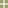The Normal CurveMain Concepts  | Demonstration  | Activity  | Teaching Tips  | Data Collection & Analysis  | Practice Questions  | MilestoneSolutions to Practice Problems 1. Many textbooks state that a value that falls more than 1.5 interquartile ranges above the upper quartile or 1.5 interquartile ranges below the lower quartile is an "outlier". What percent of the values in a normal distribution are considered "outliers" using this definition? Since the tail area for the lowest 25% has a z= -0.67 and the z for the upper 25% is z = 0.67, the interquartile range is 1.34.   1.5*1.34=2.01.  So we need to find the percent that falls above .67+2.01 = 2.68 and the percent that falls below -2.68.   The tail area is 2*0.0037 = 0.0074 = .74%. Note:  In this problem, we based our calculations for the IQR on the population distribution (the normal curve).  When making a boxplot, the IQR is based on the sample.  The sample has variability in it, also, and so in practice we identify more than .74% of our observations as outliers. 2. Imagine two outstanding students who have excelled in academics, service, and athletics. Anita earned 620 on the SAT verbal and Bonita scored 27 on the ACT. In other respects, their high school records are comparable. You learn that the average SAT verbal score is 500, with standard deviation of 100 points while the ACT has a mean of 18 with a standard deviation of 6. If we assume that scores on these exams are approximately normal, can you use thier scores to determine who wins the scholarship? Bonita wins since her score is 1.5SD's above average and Anita's score is 1.2 SD's above average. 3. Anita scored at the 60th percentile on a midterm exam while Bonita scores at the 85th percentile. If we know that the midterm had a mean of 70 and standard deviation of 10 points, how many points separate Anita and Bonita on this exam? Anita's score = 70+.26(10) = 72.6 Bonita's score = 70+1.04(10) = 80.4 The difference in scores is 80.4-72.6 = 7.8 points. 4.  National Fruit Company claims that the weights of their forty pound boxes of imported bananas are approximately normal with mean 41 pounds and standard deviation of 4 ounces. How often will a box of bananas be underweight? Since z = (40-41)/0.25 = -4, it is unlikely that any box will be underweight. 5.  For women athletes at UCLA, 62.5 inches is the 25th percentile height and 65.5 inches is the 75th percentile height. a) If we assume that heights for women athletes are approximately normal, find the mean and standard deviation of the distribution of heights. b) Use the calculations to determine the 90th percentile height for women athletes at UCLA. Using the equations 65.6 = mu + 0.67sigma and 62.5 = mu - 0.67 sigma, we have 128 = 2mu or mu = 64 inches and sigma = 2.24 inches.  Then the 90th percentile height is 64+1.28(2.24) = 66.87 inches.